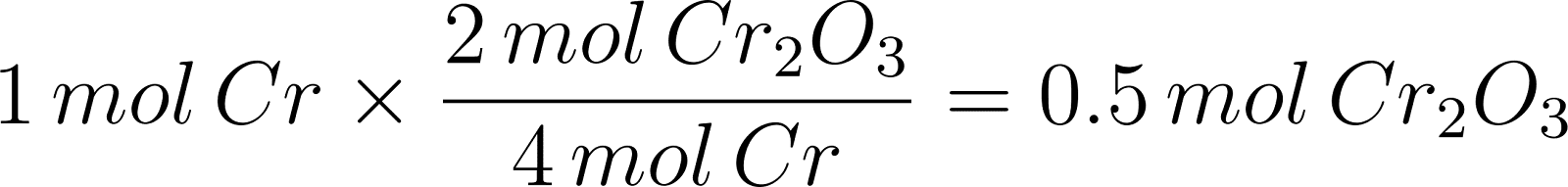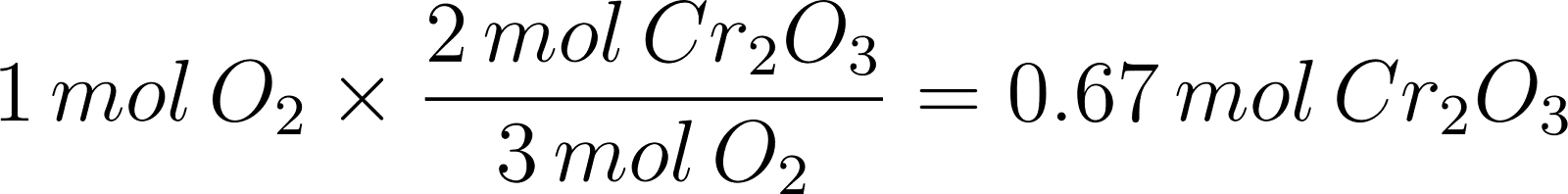×
Get Full Access to Introductory Chemistry - 5 Edition - Chapter 8 - Problem 48p
Get Full Access to Introductory Chemistry - 5 Edition - Chapter 8 - Problem 48p

×

# Solved: For the reaction shown, find the limiting reactant for each of the initialISBN: 9780321910295 34

## Solution for problem 48P Chapter 8

Introductory Chemistry | 5th Edition

• Textbook Solutions
• 2901 Step-by-step solutions solved by professors and subject experts
• Get 24/7 help from StudySoup virtual teaching assistantsIntroductory Chemistry | 5th Edition

4 5 1 312 Reviews
18
5
Problem 48P

PROBLEM 48P

For the reaction shown, find the limiting reactant for each of the initial quantities of reactants.

4 Cr(s) + 3 O2(g) → 2 Cr2O3(s)

(a) 1 mol Cr; 1 mol O2

(b) 4 mol Cr; 2.5 mol O2

(c) 12 mol Cr; 10 mol O2

(d) 14.8 mol Cr; 10.3 mol O2

Step-by-Step Solution:

Problem 48P

For the reaction shown, find the limiting reactant for each of the initial quantities of reactants.(a) 1 mol Cr; 1 mol O2

(b) 4 mol Cr; 2.5 mol O2

(c) 12 mol Cr; 10 mol O2

(d) 14.8 mol Cr; 10.3 mol O2

Step by step solution

Step 1 of 4

a)

The limiting reactant in a reaction is the reactant that produces the least amount of product.

The given chemical reaction is as follows.From the balanced chemical reaction, four moles of chromium reacts with three moles of oxygen molecules to produce two moles of chromium oxide.

Let’s calculate the number of moles of product that can be produced from one mol of “Cr” and one mol of oxygen.Hence, “Cr” produces less amount of chromium oxide.

Therefore, the limiting reactant is 1 mol Cr.

____________________________________________________________________________

Step 2 of 4

Step 3 of 4

##### ISBN: 9780321910295

Unlock Textbook Solution